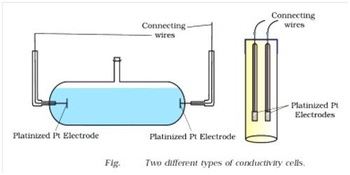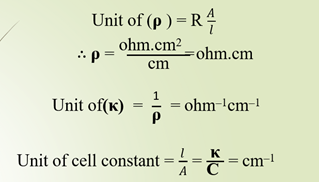# ConductatnceMetallic and electrolytic conductor:

Metallic conductors:

Those conductors which conduct electricity due to the migration of mobile free electron is known as metallic conductor. Metallic conductors are also known as electronic conductors. Conductivity of metallic conductor decreases with increase in temperature. No chemical change occurs when metallic conductor conduct electricity through them. For example, copper wire, aluminum wire , iron rod ,zinc plated etc.

Electrolytic conductors:

Those conductors which conduct electricity due to the migration of ions are called electrolytic conductors. They are also known as ionic conductors. Conductivity of electrolytic conductor increases with increase in temperature. Chemical change occurs when electrolytic conductor conduct electricity through them. For example ,fused salt aqueous solution of acid, base and salt etc.

Difference between electrolytic & Metallic Conductor:

 Electrolytic conductor Metallic conductor i.                 Electrolytic conductor conducts electricity due to the migration of ions. ii.                Conductivity of electrolytic conductor increases in increase with temperature. iii.               Chemical change occur when electrolytic conductor conduct electricity. i.                 Metallic conductor conducts electricity due to the migration of mobile free electrode.   ii.                Conductivity of metallic conductor decreases with increase in temperature. iii.               No chemical change occurs when metallic conductor conduct electricity.

Specific Resistance (ρ):

It is the reciprocal of specific conductance. It is denoted by a symbol (ρ)and also known as resistivity.Thus, specific resistance is defined as the resistance of the conductor having length 1cm and cross-sectional area 1cm2.

In case of electrolytic soln. it is the resistance of unit volume(1cc) of soln.

Specific Conductance(κ):

It is the reciprocal of specific resistance and denoted by a symbol (κ). It is also known as conductivity. Mathematically,Thus, specific conductance is κ= C. Therefore, specific  conductance is defined      as the conductance of the conductor having  length 1cm and cross sectional area 1cm2.

In case of electrolytic soln. it is the conductance of unit volume of soln        i.e. 1cc of solution.

Cell constant:

It is the ratio of the distance (l) between the electrodes to the  cross sectional area of the electrode (A) of the given conductivity cell.

Alternatively, it is defined as the ration of the specific  conductance to the observed conductance for a given electrolytic soln.Equivalent conductance (λeq ) :

Equivalent conductance of an electrolytic solution is defined as the conducting power of all the ions provided by 1 gm equivalent of an electrolyte in a certain volume of solution when the whole solution is placed between the electrode having distance 1cm and cross-sectional area of an electrode is so large that the whole solution is totally occupied between them. It is denoted by a symbol (λeq ) .

If 1gm equivalent of an electrolyte is dissolved in Vcc of solution.

Then, (λeq ) = κ×V………….. (i)

If the concentration of the solution is expressed in normality, then, for N normal solution.

N gm eq. of an electrolyte dissolved in 1000 cc of soln .

1 gm eq. of an electrolyte dissolved in1000/N cc of soln .

So, eqn (i) becomes,The unit of equivalent conductance is,

eq ) = ohm–1cm2eq–1

Molar conductance(μ):

Molar conductance of an electrolytic soln is defined as the conducting power of all the ions provided by 1mole of an electrolyte in a certain volume of soln when the whole soln is placed between the electrode having distance 1cm and cross sectional area of an electrode is so large that the whole soln is occupied between them. It is denoted by a symbol m. 4950

If 1 mole of an electrolyte is dissolved in Vcc of soln then,

m = κ×V ……(i) If the conn of the soln is expressed in morality,

For( M) molar soln .

M mol of an electrolyte dissolved in 1000 cc of soln .

1 mol of an electrolyte dissolved in 1000/M cc of soln .

So, eqn (i) becomes,

m………… (ii)

The unit of molar conductance is =Ohm-1cm2 mol-1

Relation between Equivalent conductance & Molar conductance:

From definition of molar conductance and equivalent conductance,Where,

κ = Specific conductance N = normality

M = molarity

Dividing eqn (ii) by eqn (i),Form relation between normality and molarity.

Normality x equivalent   wt. = Molarity x molecular Wt.

So equation iii becomesWhere,

Z = Acidity for Base

= Basicity for Acid

= Charge of cation or anion for salt

Variation of conductance with dilution:

i)    Specific conductance:

Specific conductance of an electrolytic soln decreases with dilution because the no. of ions per unit volume decreases with increase  in volume.

ii)    Equivalent conductance and molar conductance:

For weak electrolytic:

Equivalent/molar conductance of weak electrolyte increase with dilution because degree of ionization of weak electrolyte increase with increase in volume.

For strong electrolyte:

Equivalent/molar conductance of strong electrolyte increase with increase in volume because the distance between the ions increases and  force of attraction between the ions decreases with (increase) dilutionFig: variation of conductance with dilution